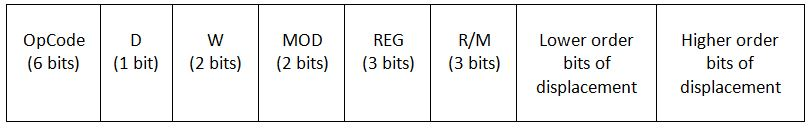# Problems: on finding the instruction format for different Instructions in 8086 Microprocessor

Here you will find some numerical problems in calculating the instruction format for different instructions. In this article, we will learn how the instruction format is formed for instruction with the help of the layout? By Monika Sharma Last updated : May 22, 2023## Question 1

Find the instruction format of the following instruction:
MOV BL , AL
(The opcode of MOV instruction is 100010)

### Solution

```We are given opcode for MOV = 100010
The value of D = 0
(as the value is being fetched from the register)

The value of W = 0
(as only a byte, i.e. 8 bits are being used)

The MOD here is 1 1
(as the mode is register mode)

The value of REG is 0 0 0	(for register AL)
The value of R/M is 0 1 1
(as the register BL is being used in the register mode)

There is no displacement present in the instruction.
So, the instruction format for the given instruction is:
10001000	11000011
```

## Question 2

The opcode of ADD instruction is 000000. Find the instruction format for the following instructions:

### Solution

```i. Given instruction is : 	ADD AX , [BX]
Here, opcode = 000000
D = 1	    (as the data is being transferred to the register)
W = 1 	    (as the processing is being done on 16 bits of data)
MOD = 0 0 	(because [BX] represents Memory mode with no displacement)
REG = 0 0 0	(as AX register is being used in the instruction)
R/M = 1 1 1	(for [BX] being used with the addressing mode)

And, there is no displacement here in this instruction,
so the remaining bits will remain empty in the instruction format.

Hence the instruction format for the given instruction is:
00000011 00000111
```
```ii. Given instruction is : 	ADD AL , [2000H]
Here, opcode = 000000 (for ADD instruction)
D = 1	    (as the data is being transferred to the register)
W = 0 	    (as the processing is being done on 8 bits of data)
MOD = 0 0 	(because [BX] represents Memory mode with no displacement)
REG = 0 0 0	(as AX register is being used in the instruction)
R/M = 1 1 0	(for direct mode being used as the addressing mode)

And the displacement here is 2000H
So, the lower bits of displacement are 00H i.e. 0000 0000
And the higher bits of displacement are 20H, i.e. 0010 0000

Hence the instruction format for the given instruction is:
00000010 00000110 00000000 00100000
```